# 怎么表示

## 数学中的数

• 数学中的数是怎么表示的，

• 有两种方法，一种是普通计数如$12345$和$123.45$，还有一种是科学计数法，如$1.2345\times10^4$和$1.2345\times10^{-2}$

• 维基

科学记数法（英语：Scientific notation，英国则称为 Standard form），又称为科学记数法科学记法，是一种数字的表示法。科学记数法最早由阿基米德提出。

• 科学记数法有什么好处

• 非常大数和非常小数表示时好处非常明显，可以很清晰看出量级和大小，如$123450000000000000000=1.2345\times10^{20},0.00000000012345=1.2345\times10^{-10}$

• 当我们要表示非常大或非常小的数时，如果用一般的方法，将一个数的所有位数都写出来，会很难直接确知它的大小，还会浪费很多空间。但若使用科学记数法，一个数的数量级精确度数值都较容易看出，例如于化学里，以公克表示一个质子质量的数值为:0.00000000000000000000000167262158, 但如果将它转成科学记数法的形式，便可不需要写那么多零︰$1.67262158\times 10^{-24}$，又例如，若以公斤为表示单位，则木星的质量值约为：1898130000000000000000000000

像这样的大数亦无法直接用列出所有位数的方式表达出精确度，但科学记数法就能用下方形式明白的表示出来：

$1.89813\times 10^{27}$

## 计算机中的数

• 计算机中的数也是类似，我们可以有多种存放方法，

• 从这里开始下面就只讨论小数（浮点数）的存放方法

• 比如0.00000000000000000000000167262158，可以先存数值本身即0 00000000000000000000000167262158，再存一个1表示小数点在哪，在第一个0的后面

• 除了上面的存放方法还有其他存放方法，计算机发展初期对于小数还有各种各样的存法，各家制定各家规则的，互相不兼容，写程序还要写几套很麻烦，后来IEEE选择一个较好的制定成标准，以后大家都别各自搞各自的了，都按照这个来，这个标准就是IEEE754二进制浮点数算术标准

## IEEE754转化过程

• IEEE754存储浮点数有点类似于十进制的科学记数法表示数

313029282726252423222120191817161514131211109876543210
s=signe=exponent(8 bits)f=fraction(23 bit)

$(-1)^{s} \ 10^{e-1111111}\times 1.f=(-1)^{s} \ 2^{e-127}\times 1.f$

$(-1)^{s} \ 10^{1-1111111}\times 0.f=(-1)^{s} \ 2^{1-127}\times 0.f$

### 规格数(10.5存储为例)

#### 规范化

##### 转二进制
• $10.5_{(10)}=1010.1_{(2)}$
##### 转二为底的科学记数法形式
• $1010.1_{(2)}=1.0101_{(2)}\times2_{(10)}^{3_{(10)}}$

• $3_{(10)}$为指数

• 为偏移前指数

• $1.0101_{(2)}$为尾数

• 必须保证尾数的第一位不是零，且小数点在第一位和第二位之间

十进制第一位不是0可以是1-9，而二进制第一位不是零只能是1

#### 性质符号填充

• 最高位填充符号，正填充0，负填充1
• 10.5是正数填充0
0
^
313029282726252423222120191817161514~3210
signexponent(8 bits)fraction(23 bit)

#### 尾数转化填充

##### 隐藏高位1和点
• $1.0101\to 0101$

• 既然尾数第一位不能是0只能是1，大家都是1和点，也没有写的不要了都省略也行

##### 低位补零
• $0101\to 0101 0000 0000 0000 0000 000$

• 补齐23位

##### 填充尾数
• 讲补齐后尾数填充在低23位上
0010100000...000
^^^^^^^^^ all 0^^^
313029282726252423222120191817161514~3210
signexponent(8 bits)fraction(23 bit)

#### 指数转化填充

##### 偏移前的指数加偏移量
• $3_{(10)}+127_{(10)}=130_{(10)}$

• 在32位单精度类型中，这个偏移量是127

在64位双精度类型中，偏移量是1023

##### 偏移后的指数转二进制
• $130_{(10)}=1000 0010_{(2)}$
##### 填充指数
• 讲补齐后尾数填充在性质符号位后的高8位上
010000010010100000...000
^^^^^^^^
313029282726252423222120191817161514~3210
signexponent(8 bits)fraction(23 bit)

#### 测试结果

• 测试网站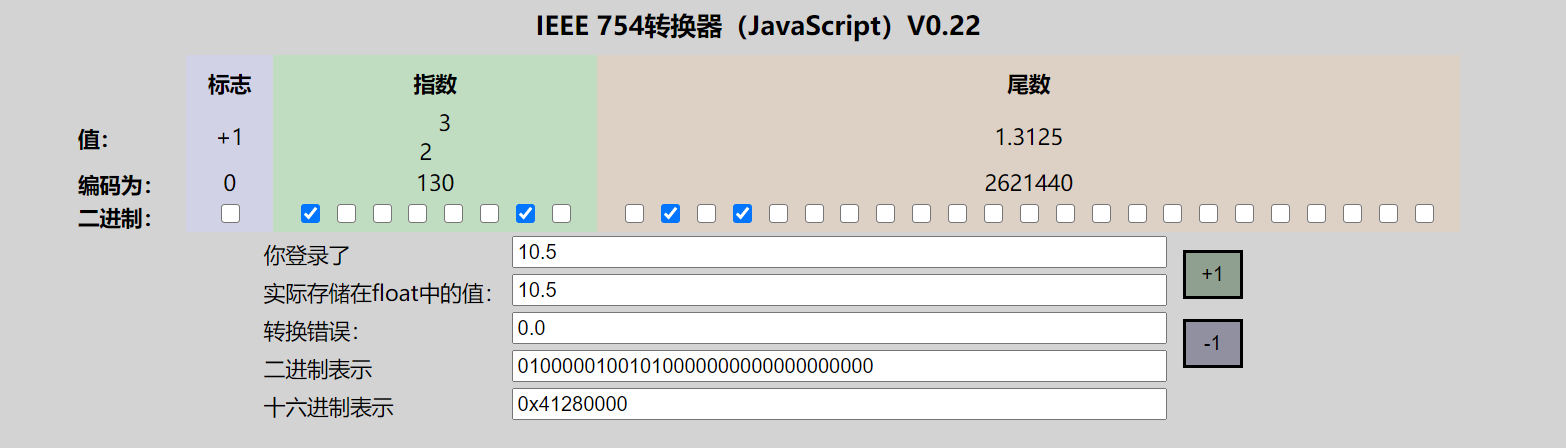### 规格数读取(10.5为例子)

#### 判断是不是规格数

• 除了符号位的高八位不全为0或者1，是规格数

• 为$sign \ 2^{exponent}\times fraction$形式

• 看其除了符号位的高八位是不是都是0是不是都是1

• 如果不都是0且不都是1，则规格化的数
• 如果都是0，则为非规格化的数
• 如果都是1
• 如果后面低23位全为0，则为无穷大
• 如果后面低23位不全为0，则为NaN，not a number
010000010010100000...000
^^^^^^^^
313029282726252423222120191817161514~3210
signexponent(8 bits)fraction(23 bit)

#### 拆分符号位

• 符号位为0为正的,代入式子

• $sign \ 2^{exponent}\times fraction=+ \ 2^{exponent}\times fraction$

• 最高位为，符号位0是正的，1是负的

010000010010100000...000
^
313029282726252423222120191817161514~3210
signexponent(8 bits)fraction(23 bit)

#### 拆分指数部分

##### 读取指数部分
• 1000 0010

• 除了符号位的高八位

010000010010100000...000
^^^^^^^^
313029282726252423222120191817161514~3210
signexponent(8 bits)fraction(23 bit)
##### 指数部分转10进制偏移后指数
• $10000010_{(2)}\to 130_{(10)}$
##### 减去偏移量转偏移前指数
• $130_{(10)}-127_{(10)}=3_{(10)}$
##### 代入式子
• $+ \ 2_{(10)}^{exponent}\times fraction=+ \ 2_{(10)}^{3_{(10)}}\times fraction$

#### 拆分尾数部分

##### 读取低23位
• 0101 0000 0000 0000 0000 000
010000010010100000...000
^^^^^^^^^^^^
313029282726252423222120191817161514~3210
signexponent(8 bits)fraction(23 bit)
##### 去掉尾部0
• 0101 0000 0000 0000 0000 000 => 0101
##### 补充高位1和点
• $0101_{(2)}\to 1.0101_{(2)}$
##### 代入式子
• $+ \ 2_{(10)}^{3_{(10)}}\times 1.0101_{(2)}$

#### 转化成科学记数法或者普通形式

• $+ \ 2_{(10)}^{3_{(10)}}\times 1.0101_{(2)}=1010.1_{(2)}$
• $1010.1_{(2)}=10.5_{(10)}$

### 非规格数(二进制读取为例)

000000000011000000...000
313029282726252423222120191817161514~3210
signexponent(8 bits)fraction(23 bit)

#### 判断是不是非规格数

• 除符号位为的高八位全部为0，是非规格数
• 也是$sign \ 2^{exponent}\times fraction$形式
000000000011000000...000
^^^^^^^^
313029282726252423222120191817161514~3210
signexponent(8 bits)fraction(23 bit)

#### 读取符号位

• 符号位为0，为正
• $+ \ 2^{exponent}\times fraction$

#### 代入指数

• 非规格数指数固定为$1-127_{(10)}=-126_{(10)}$

• 非规格数指数是 1-偏移量 （而不是$0-127=-127$）

这是规定

规格数指数才是 实际指数-偏移量

• $+ \ 2_{(10)}^{-126_{(10)}}\times fraction$

#### 读取代入尾数

##### 读取尾数
• 0110 0000 0000 0000 0000 000
000000000011000000...000
^^^^^^^^^^^^
313029282726252423222120191817161514~3210
signexponent(8 bits)fraction(23 bit)
##### 去掉尾部0
• $0110 0000 0000 0000 0000 000_{(2)}\to011_{(2)}$
##### 补充0.
• $0110_{(2)}\to 0.011_{(2)}$

• 非规格数补充0.

而规格数补充1.

##### 代入式子
• $+ \ 2_{(10)}^{-126_{(10)}}\times fraction\to + \ 2_{(10)}^{-126_{(10)}}\times 0.011_{(2)}$

#### 转化成普通形式

• $+ \ 2_{(10)}^{-126_{(10)}}\times 0.011_{(2)}\to + \ 2_{(10)}^{-126_{(10)}}\times 0.375_{(10)}\to 4.408103815583578155e-39$

#### 测试结果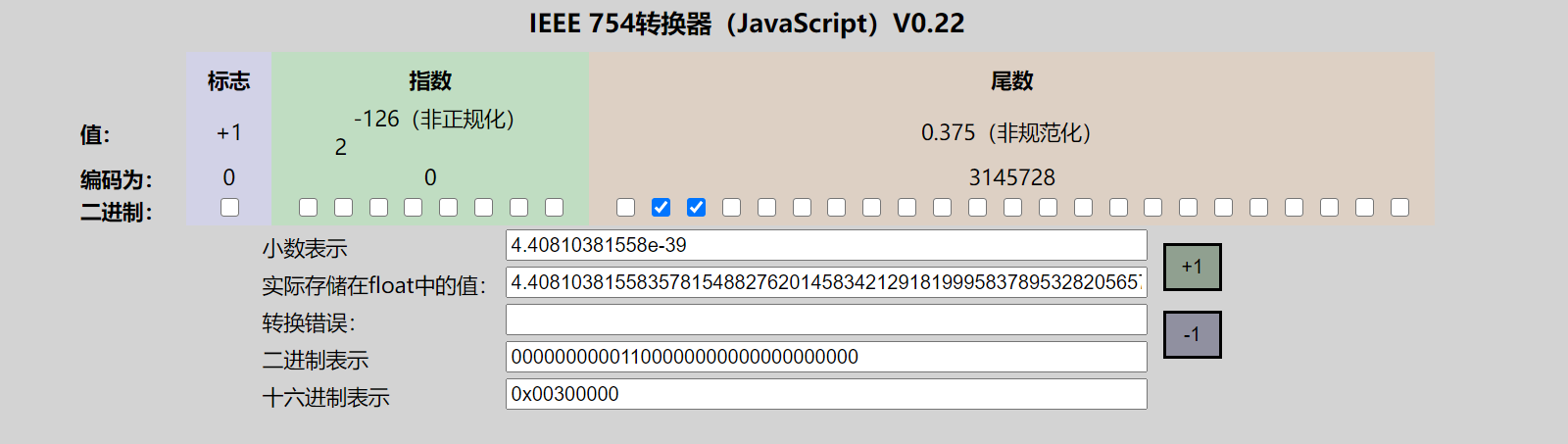### 特殊数

• 最后一类数值是当指阶码（指数部分）全为1 的时候出现的。
• 当小数域全为0时， 得到的值表示无穷，
• 当符号位为0时是正无穷 ,
• 或者当符号位为1时是负无穷。
• 当我们把两个非常大的数相乘， 或者除以零时， 无穷能够表示溢出的结果。
• 当小数域为非零时， 结果值被称为”NaN”, 即 “不是一个 数(Not a Number)” 的缩写。
• 一些运箕的结果不能是实数或无穷， 就会返回这样的NaN 值，比如当计算$\sqrt{-1}$或$\infty-\infty$时。 在某些应用中，表示未初始化的数据时，它们也很有用处。
• 这个不区分正负，都是NaN

#### 无穷大

011111111000000000000

313029282726252423222120191817161514~3210
signexponent(8 bits)fraction(23 bit)
111111111000000000000

313029282726252423222120191817161514~3210
signexponent(8 bits)fraction(23 bit)

#### NaN

*11111111************
NaN^ 都是1^ *代表0或1都可以
313029282726252423222120191817161514~3210
signexponent(8 bits)fraction(23 bit)

# 范围与精度

## IEEE754

32位float

### 范围

#### 影响因素

• 主要由指数部分决定
010000010010100000...000
^^^^^^^^
313029282726252423222120191817161514~3210
signexponent(8 bits)fraction(23 bit)
#### 范围

+0000000000.0
–080000000–0.0
13f8000001.0
2400000002.0

+∞7f800000正无穷
–∞ff800000负无穷

NaN（Not a Number, 非数）可以用任何满足 NaN 定义的位模式表示。在上表中显示的 NaN 十六进制值只是可用于表示 NaN 的众多位模式之一。

+∞7f800000正无穷
NaN大于最大正规数且不为正无穷由于存储表示非数字，就无法表示数

eg普通正的正规数400000002.0中间的数也不一是连续的，是离散的且涉及精度

eg普通的次正规数0x00600000$8.81620763117e-39$非正规数有时候不归在float可表示的数里

err小于最小次正规数且大于正0无穷小的数无法表示的数
+0000000000.0
–080000000–0.0
err小于0且大于最大次负规数无穷小的数无法表示的数

eg普通的负次正规数0x80600000$-8.81620763117e-39$

eg普通负的正规数0xcc189680$-40000000.0$

NaN小于最小正规数且不为负无穷0x80189680$-2.25804113503e-39$无法表示的数
–∞ff800000负无穷
• 规格数，（正的负的都是）最大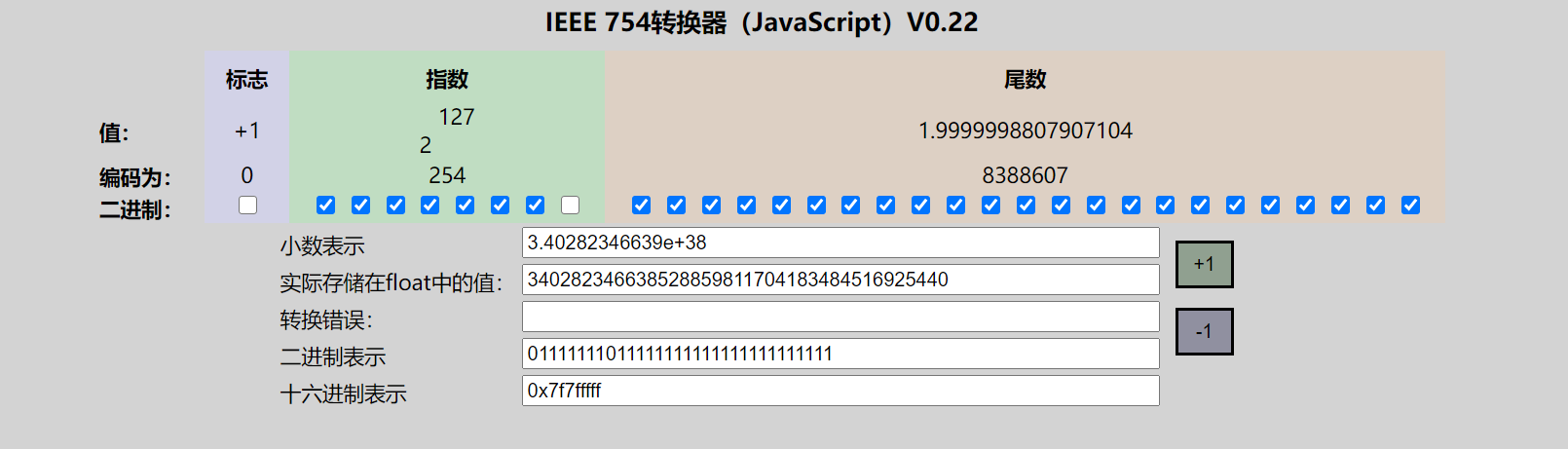• 规格数，（正的）最小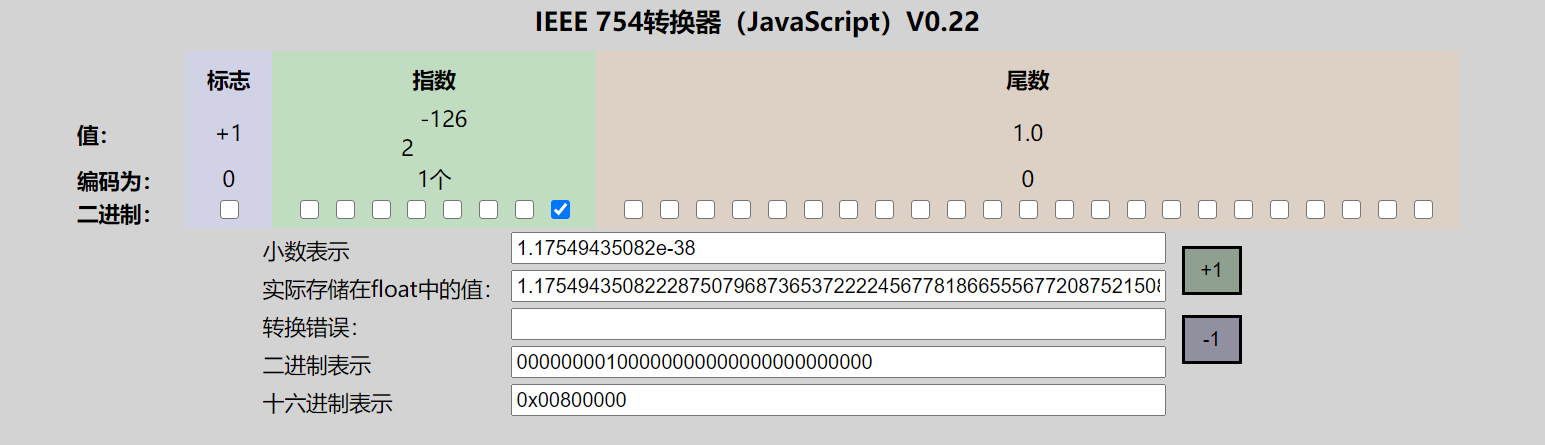• 规格数，（负的）最大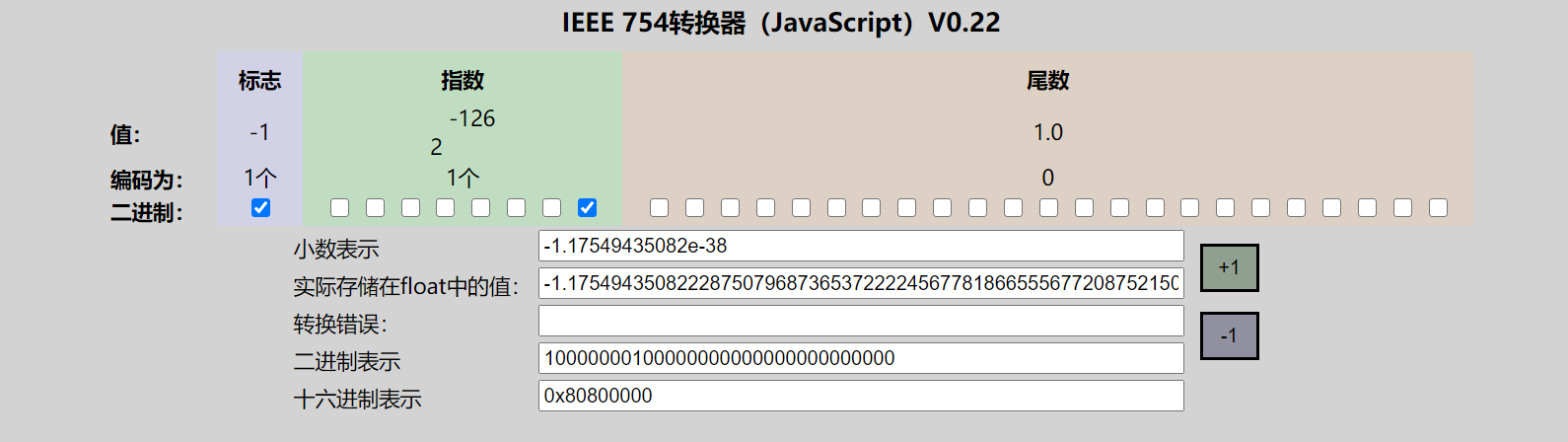• 规格数，（正的负的都是）最小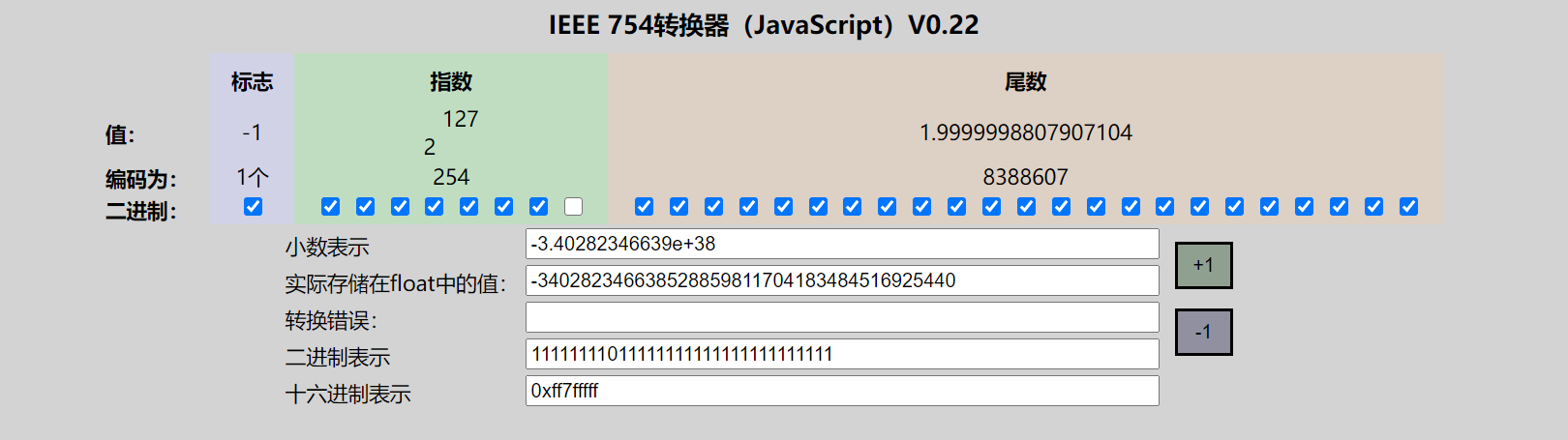• 次规格数，（正的负的都是）最大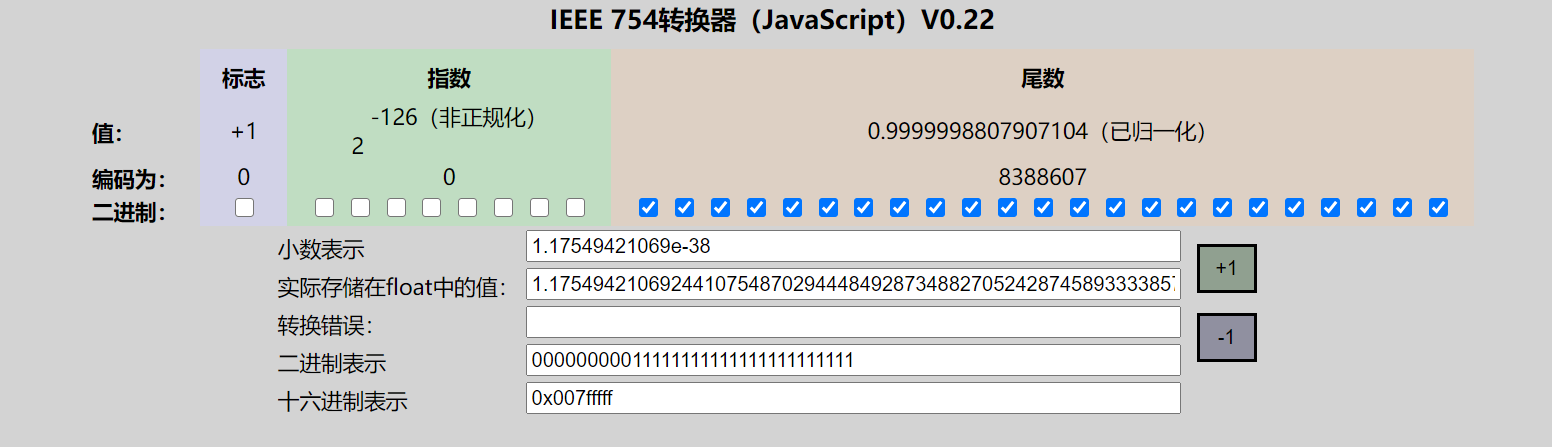• 次规格数，（正的）最小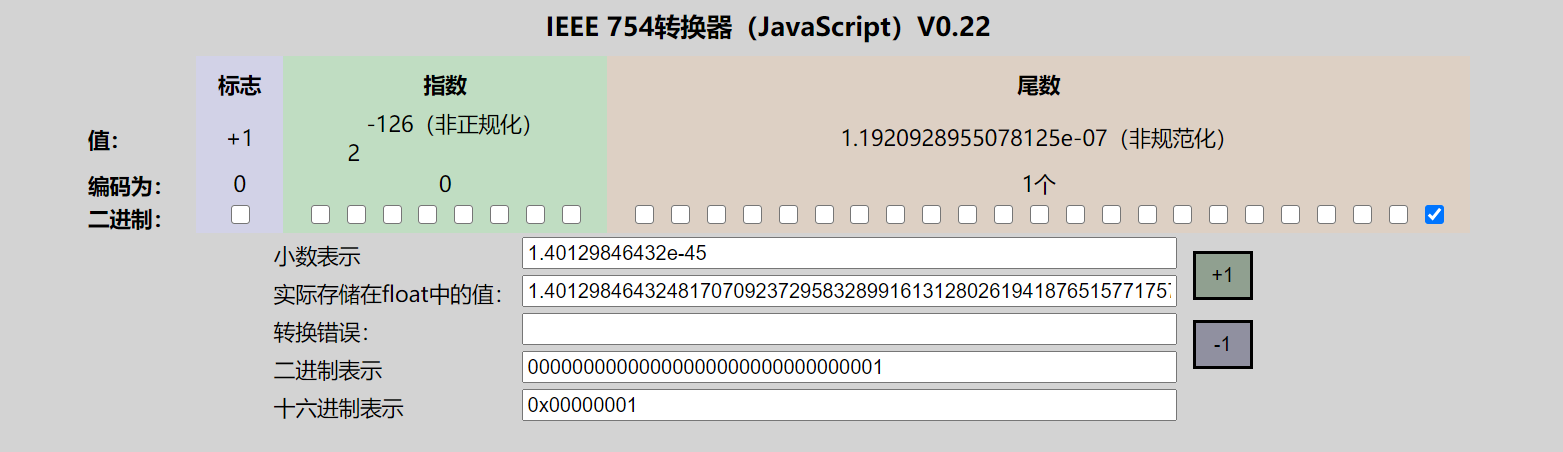• 次规格数，（负的）最大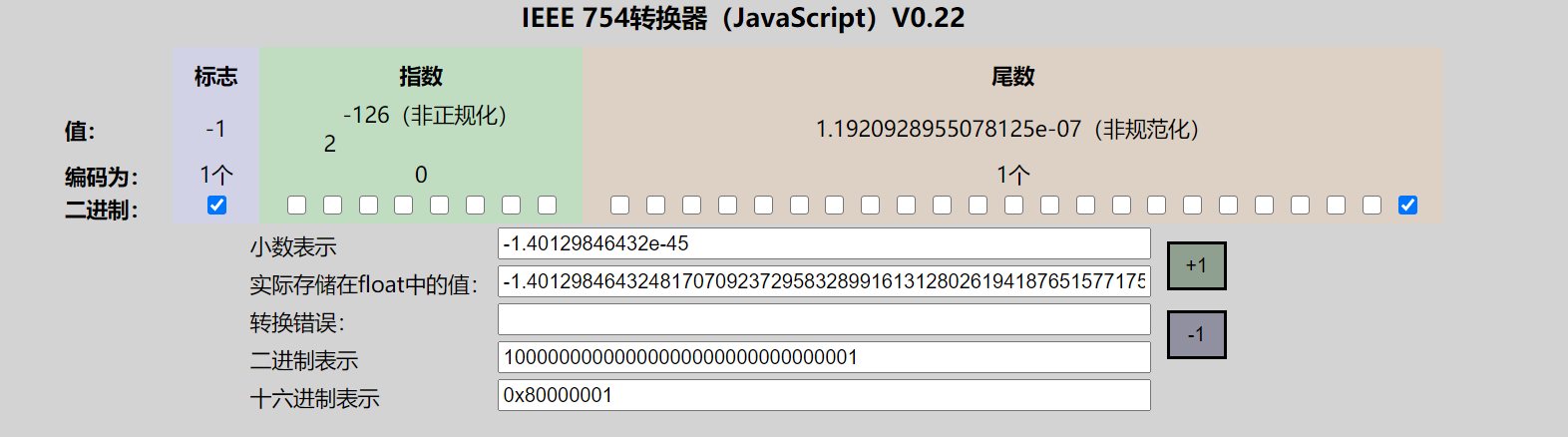• 次规格数，（正的负的都是）最小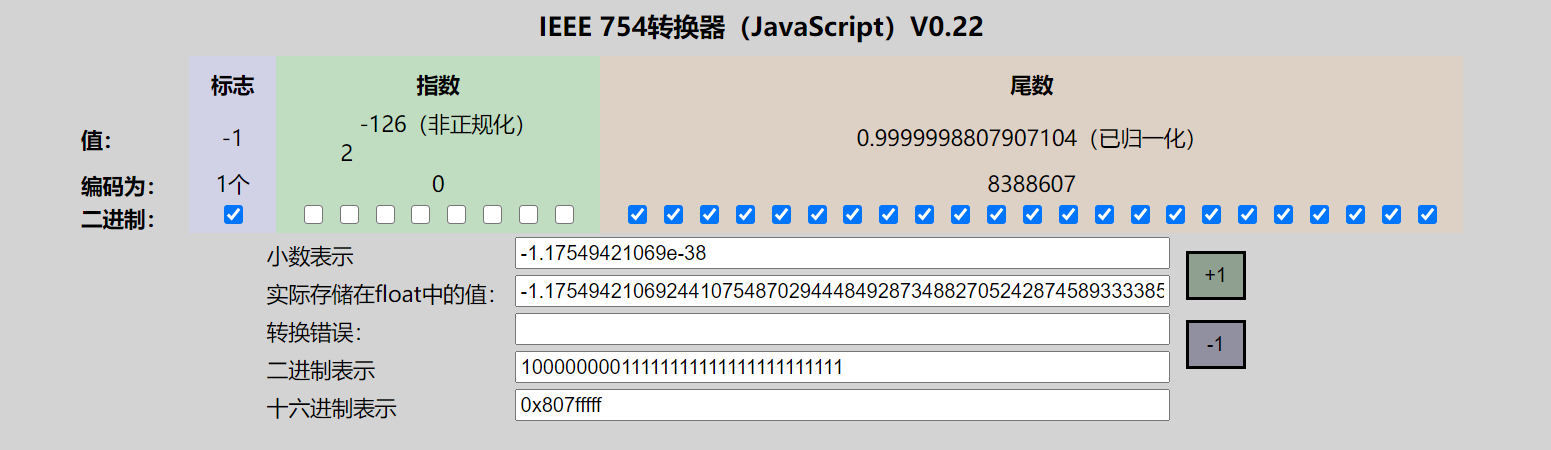### 精度

• $2^{23}=8388608$

• 这么多组合，可以表示这么多不同的数,8388608这个一个7位数，6位是准的，7位不一定准

$2^{52}=4503 5996 2737 0496$是一个16位数，至少保证15位数存储是准的，至少可能存储15位有效数字

### 误差

• 十转二误差
• 不是所有都可以表示为$\sum_{i=1}^{n}[0|1]\times2^{N_i},(N_i\in \text{整数},n\neq\infty)$
• 如$0.000110011001……$存储时会截断存储产生误差

• 不能准确存储下列
• 存储无穷大

• 和无穷小量(包括 自然数+无穷小量 形式)，

• 即有限的空间本身就是只能存储有限的离散的点

• 运算时产生无法准确存储的数据